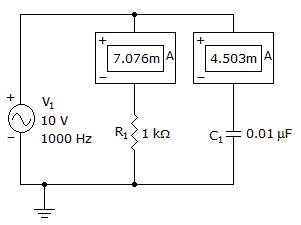# Electronics - Capacitors - Discussion

### Discussion :: Capacitors - General Questions (Q.No.51)

51.

What is the circuit's impedance?[A]. 1192[B]. 843[C]. 723[D]. 511Explanation:

No answer description available for this question.

 Sumit Aole said: (Dec 20, 2011) v=10; total current I =sqrt(Ic^2+Ir^2); I = sqrt(7.076*10*^-3^2+ 4.608*10*^-3^2); I= 8.444*10^-3 z = 10/8.444*10^-3= 1184.25~~1192;

 Yogita Lakhi said: (Aug 24, 2014) If we solve it using Z=sqrt (R^2+Xc^2), the answer is different.

 Sivapramod said: (Sep 1, 2014) No. This is a parallel RC Circuit, So use the below formula, Z = RXc/sqrt(R^2+Xc^2).

 Lana said: (Oct 11, 2015) Can anyone please show the correct solution for this? Thank you!

 Sumeet said: (Sep 10, 2016) Total current = square root(Ic^2 + Ir^2). Z = Voltage/total current = 10/total current.